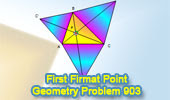## Friday, May 14, 2010

### Geometry Problem 903: First Fermat Point

Problem 903. Post your solution in the comments box below
Triangle centers

A triangle with three equilateral triangles erected externally on the sides.
Click the figure below to see the complete illustration.See also:
Fermat Point
Level: High School, SAT Prep, College geometry

#### 3 comments:

1.in triangles AC'C and ABB', AC'= AB, AB'= AC because triangles ABC' and AB'C are equilateral. also angle C'AC = angle BAB' as angle BAC is common to both and angle C'AB = angle B'AC = 60 degrees because the triangles ABC' and ACB' are equilateral. so by SAS criterion the triangles AC'C and ABB' are congruent. this implies that AC'F = angle ABF. but AF being the common segment to both. so these angles are in fact in the same segment if a circle is drawn to circumscribe the quadrilateral AFBC'. that is AFBC' is a cyclic quadrilateral. similarly the quadrilaterals A'BFC and AB'CF are also cyclic. therefore angle AFC = angle AFB = angle BFC as the angles AC'B, AB'C and BA'C are each equal to 60 degrees because the corresponding triangles are equilateral. we know that the opposite angles of a cyclic quadrilateral are supplementary, so angle AFC = angle AFB = angle BFC = 120 degrees. now notice let us assume that CC' and BB' intersect at F. we have to show that AA' is a straight line o prove that the segments AA', BB' and CC' are concurrent. notice that angle AFB = 120 degrees and angle A'FB = angle A'CB as the quadrilateral A'BFC is cyclic and these angles are in the same segment. so angle A'FB = angle A'CB = 60 degrees because triangle BA'C is equilateral. angle A'FB + angle AFB = 120 + 60 = 180 degrees. this shows that these angles constitute a linear pair. so AA' is a straight line which indeed passes through F. these prove the first and second parts of the theorem. again notice that A'BFC is cyclic. let us choose another point say F' other that F. then by ptolemy's inequality, we get BF'.A'C + BA'.F'C ≥ A'F'.BC we know that A'B = BC = A'C so the inequality reduces to BF' + CF' ≥ A'F'. but we knoe that F lies on the circle so the inequality now reduces to equality implying BF + CF = A'F. this also implies that BF + CF + AF = A'F + AF = AA'. for any other point F we have by the inequality that BF' + CF' + AF' ≥ A'F' + AF'. this shows that AF + BF + CF is the minimum possible. also by the congruence that was derived we get AF + BF + CF = AA' = BB' = CC'. this proves the third part also.
Q. E. D.

2.how was it? please give a reply

3.http://img706.imageshack.us/img706/1367/8bq.png
Questions 1 and 2 already had solutions (see problem 404)
Below is the solution to question 3:
Let F’ is any point inside triangle ABC and denote d(F’)=F’A+F’B+F’C
Draw equilateral triangles CF’E’ and CFE ( see sketch)
Since ∠ (CFB’)= ∠ (CFE)=60 => E will be on FB’
Note that ∆ (CF’A) and ∆ (CE’B’) are congruence … ( case SAS) => F’A=E’B’
So d(F’)= F’A+F’C+F’B=F’A+F’E’+F’B’.
Between points A and B’ , straight line is the shortest distance
So AB’ ≤ d(F’)
Equal sign only happen when F’ coincide to F , in this case E’ coincide to E
So F is the point where distance FA+FB+FC is minimum.